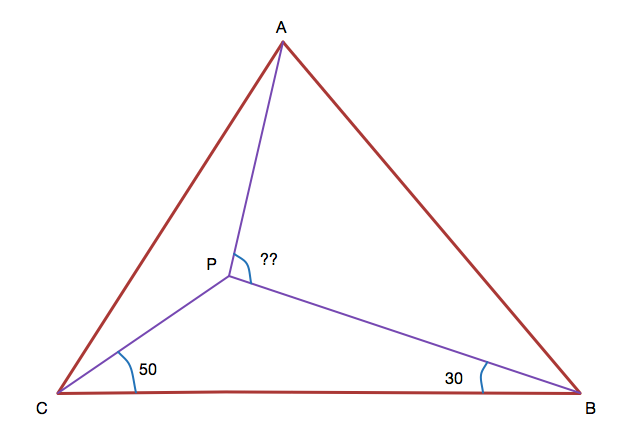# A geometry problem by sujoy roy

Geometry Level 4ABC is an isosceles triangle with AB=AC and $\angle BAC=40^{\circ}$. P is a point inside the triangle such that $\angle PBC=30^{\circ}$ and $\angle PCB=50^{\circ}$. Find $\angle APB$.

Note: Use only geometry to solve the problem.

×